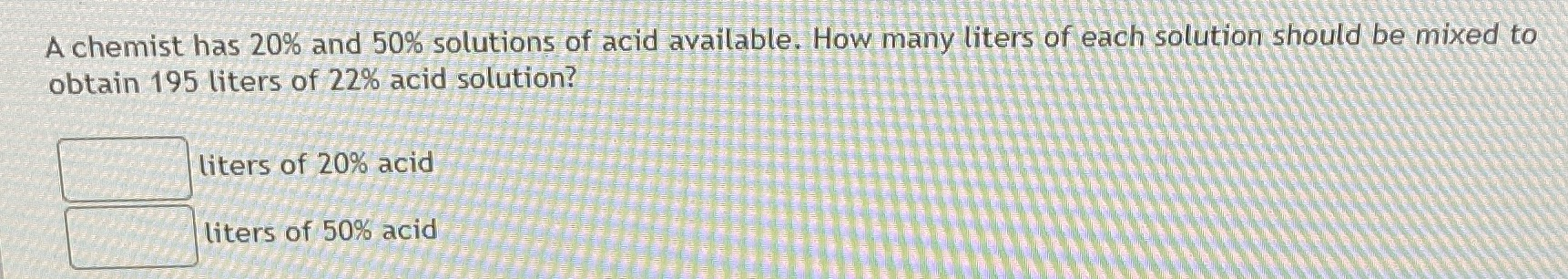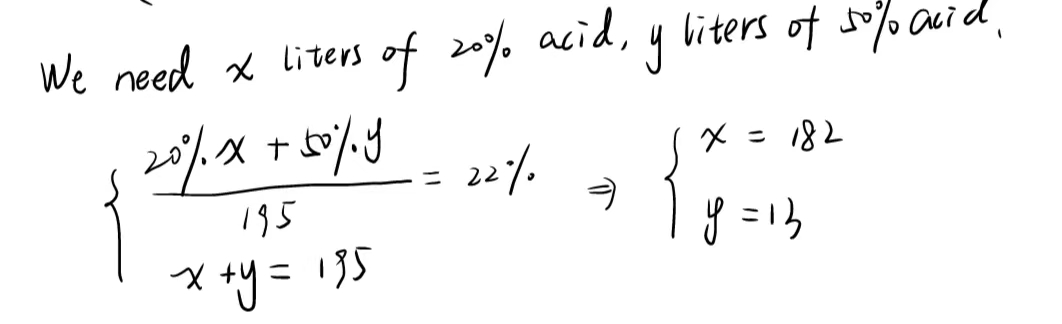### ¿Todavía tienes preguntas de matemáticas?

Pregunte a nuestros tutores expertos
Algebra
PreguntaA chemist has $$20 \%$$ and $$50 \%$$ solutions of acid available. How many liters of each solution should be mixed to obtain $$195$$ liters of $$22 \%$$ acid solution? liters of $$20 \%$$ acid liters of $$50 \%$$ acid